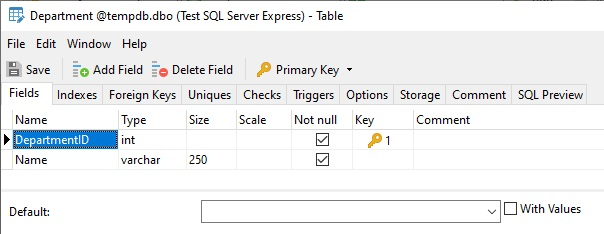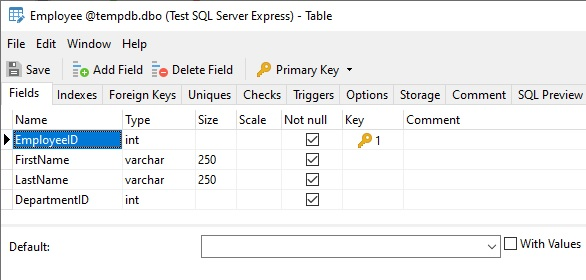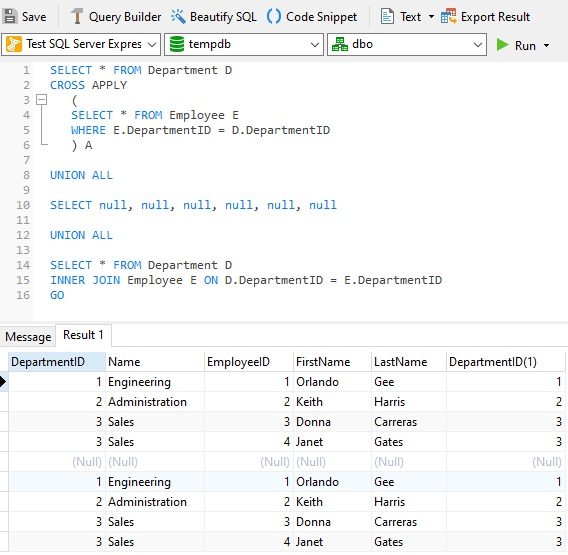Navicat Blog

Understanding SQL Server CROSS APPLY and OUTER APPLY Queries - Part 1 Sep 27, 2021 by Robert Gravelle

Part 1: APPLY vs JOIN

As you are probably aware, JOIN operations in SQL Server are used to join two or more tables. However, in SQL Server, JOIN operations cannot be used to join a table with the output of a table valued function. In case you have not heard of table valued functions, these are functions that return data in the form of tables. In order to allow the joining of two table expressions SQL Server 2005 introduced the APPLY operator. In this blog, we'll learn how the APPLY operator differs from regular JOINs.

About CROSS APPLY and OUTER APPLY

SQL Server APPLY operator comes in two variations: CROSS APPLY and OUTER APPLY:

• The CROSS APPLY operator returns only those rows from the left table expression (in its final output) if it matches with the right table expression.
Thus, the CROSS APPLY is similar to an INNER JOIN, or, more precisely, like a CROSS JOIN with a correlated sub-query with an implicit join condition of 1=1.
• The OUTER APPLY operator returns all the rows from the left table expression regardless of its match with the right table expression. For those rows for which there are no corresponding matches in the right table expression, it returns NULL values in columns of the right table expression.
Hence, the OUTER APPLY is equivalent to a LEFT OUTER JOIN.

Although the same query can be written using a normal JOIN, the need of APPLY arises when you have a table-valued expression on the right side and you want this table-valued expression to be evaluated for each row from the left table expression. Moreover, there are cases where the use of the APPLY operator can boost query performance.

Let's explore the APPLY operator further with some examples.

The Sample Data

We'll execute our queries against two new tables that we'll create in Navicat for SQL Server. Here is the design for the Department table:Here is the design for the Employee table:Execute the following SQL in the Navicat Query Editor to populate the tables:

INSERT [Department] ([DepartmentID], [Name])
VALUES (1, N'Engineering')
INSERT [Department] ([DepartmentID], [Name])
INSERT [Department] ([DepartmentID], [Name])
VALUES (3, N'Sales')
INSERT [Department] ([DepartmentID], [Name])
VALUES (4, N'Marketing')
INSERT [Department] ([DepartmentID], [Name])
VALUES (5, N'Finance')
GO

INSERT [Employee] ([EmployeeID], [FirstName], [LastName], [DepartmentID])
VALUES (1, N'Orlando', N'Gee', 1 )
INSERT [Employee] ([EmployeeID], [FirstName], [LastName], [DepartmentID])
VALUES (2, N'Keith', N'Harris', 2 )
INSERT [Employee] ([EmployeeID], [FirstName], [LastName], [DepartmentID])
VALUES (3, N'Donna', N'Carreras', 3 )
INSERT [Employee] ([EmployeeID], [FirstName], [LastName], [DepartmentID])
VALUES (4, N'Janet', N'Gates', 3 )

CROSS APPLY vs INNER JOIN

Here is a query that is made up of two parts: the first query selects data from the Department table and uses a CROSS APPLY to evaluate the Employee table for each record of the Department table; the second query simply joins the Department table with the Employee table to produce the same results:Coming up in Part 2

Having introduced the APPLY operator in this blog, Part 2 will outline the differences between using APPLY and JOIN and provide additional uses for APPLY.

Blog Archives
Share
• Key Topics• Products• Partners